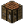# LoopsTutorial: Loops This is a tutorial page for loops and their application. Objective To understand the purposes, and uses of, loops and iterators Prerequisites A basic understanding of Variables and a basic understanding of Functions.

## Introducing Loops

At some point while coding, you will most likely want to run some code multiple times, without writing out a lot of code. While it is true that functions can shorten your code, there may be situations where even those don't simplify it enough. That's where loops come in.

Here is a name-gathering script without loops:

```local function GetName()
write("Enter a name: ")
end

local Name1 = GetName()
local Name2 = GetName()
local Name3 = GetName()
local Name4 = GetName()
local Name5 = GetName()

write("You have entered "..Name1.." "..Name2.." "..Name3.." "..Name4.." "..Name5)
```

As you can see, there is quite a lot of repetition, even when we're using a function.

## The For loop

The for loop is the most basic loop. It is in the following format:

```for Variable = Start, End, Interval do
--Insert code here
end
```

Variable is a number that will track where we are in the loop. It is usually called "i", or something more appropriate to the script. Start is a number to indicate the start point for the loop. In a simple loop this will typically be 1, but you may have need to start with other values. End is the number the loop will count to. Interval is an optional argument, and will specify how much to increment (or decrement!) Variable by with each iteration.

The best way to discover how the for loop works is to try it yourself. Try changing the numbers the following code to see how it reacts.

```for i=1,10 do
print( "i is "..tostring(i) )
end
```

Notice how it always counts up until Variable is the same or greater than End. Start, End, and Interval can also be variables, so it can loop a different number of times:

```write("How many times should I loop? ")
local Num = tonumber( read() )
for i=1,Num do
print("Looped "..tostring(i).." time(s).")
end
```

Note that the loop only inspects Start, End, and Interval once - when the loop starts - so if their values change while the loop is running, it'll ignore this and loop according to as they were when it it begun.

To simplify the first script using a for loop, it would look something like this:

```local string = ""
for i=1,5 do
write("Enter a name: ")
end
write("You have entered "..string)
```

A for loop's code will not be executed at all if you hand it values such that the loop appears to've already ended. For example:

```for i=1,0 do
print(i)
end
```

The above code will print nothing, as Start starts out as above End.

## The While loop

The While loop will continue looping until a certain condition is no longer met.

```local Answer, Correct = "", "2"
while Answer ~= Correct do
write("What is the sum of 1 and 1 (1+1)? ")

if Answer == Correct then print("Correct!") else print("Incorrect!") end
end
```

This type of loop is useful for if you don't want to specify a definite end for the loop. Although infinite loops are ill-advised, you may want to use one at some point. To terminate a program stuck in a loop, hold Ctrl + T while in the computer's interface.

```while true do --Always loop
--Code here
end
```

## The Repeat loop

The reverse of a while loop - instead of repeating so long as a condition is true, it repeats until a condition is true. Since the check is done at the bottom of the code block, the code within it will always be executed at least once.

```local i = 1
repeat
print(i)
i = i + 1
until i == 5
```